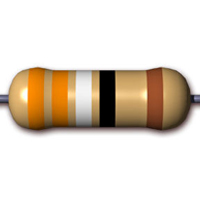# 5 Band Resistor Color Code Calculator

5 Band Resistor Color Code Tool: This tool is used to calculate the information for color banded axial lead resistors of 5 band. Select the number of bands, then their colors to determine the value and tolerance of the resistors.

#### 5 Band Resistor Color Code Description

The number of bands is important because the decoding changes based upon the number of color bands. For the 5 band resistor:

Band 1 – First significant digit

Band 2 – Second significant digit

Band 3 – Third significant digit

Band 4 – Multiplier

Band 5 – Tolerance

#### Formulae for calculating resistance of a 5-band resistor

Resistance = (First Significant Digit . Second Significant Digit. Third Significant Digit) * Multiplier ± Tolerance %